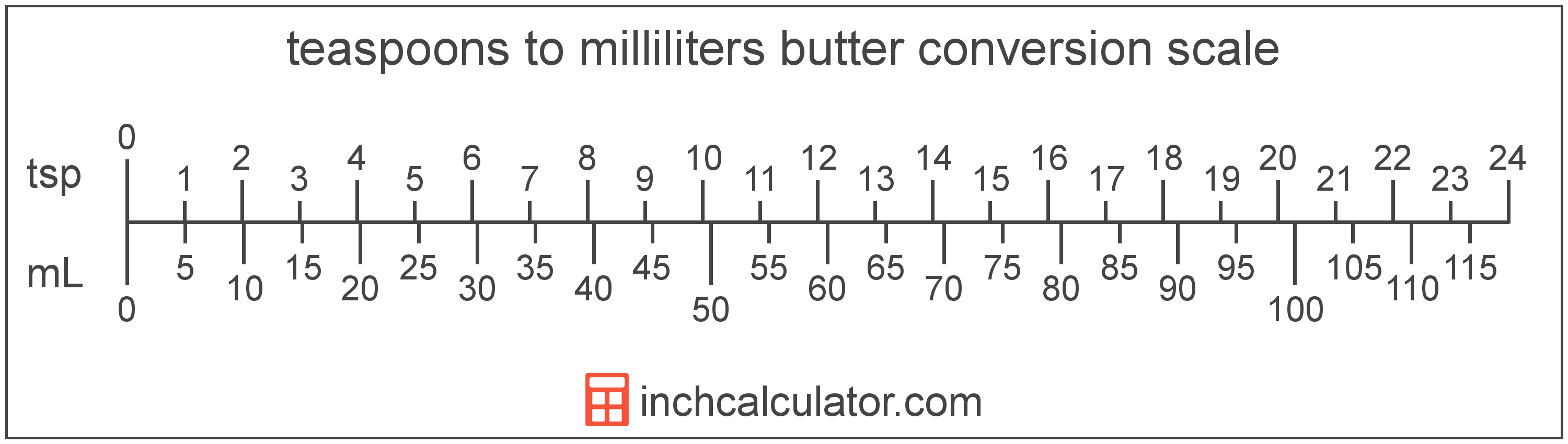# Convert Milliliters of Butter to Teaspoons

Enter the butter in milliliters below to get the value converted to teaspoons.

Results in Teaspoons:1 mL = 0.202884 tsp

Do you want to convert teaspoons to milliliters?

## How to Convert Milliliters to Teaspoons

To convert a measurement in milliliters to a measurement in teaspoons, multiply the butter by the following conversion ratio: 0.202884 teaspoons/milliliter.

Since one milliliter of butter is equal to 0.202884 teaspoons, you can use this simple formula to convert:

teaspoons = milliliters × 0.202884

The butter in teaspoons is equal to the butter in milliliters multiplied by 0.202884.

For example, here's how to convert 5 milliliters to teaspoons using the formula above.
teaspoons = (5 mL × 0.202884) = 1.014421 tsp### How Many Teaspoons Are in a Milliliter of Butter?

There are 0.202884 teaspoons in a milliliter of butter, which is why we use this value in the formula above.

1 mL = 0.202884 tsp

## What is a Milliliter?

Butter is sometimes measured by volume in milliliters. One stick of butter contains about 118.3 milliliters.

The milliliter is an SI unit of volume in the metric system. A milliliter is sometimes also referred to as a millilitre. Milliliters can be abbreviated as mL; for example, 1 milliliter can be written as 1 mL.

## What is a Teaspoon?

One teaspoon of butter is equal to 1/3 of a tablespoon or about 4.7 grams.

The teaspoon is a US customary unit of volume. Teaspoons can be abbreviated as tsp, and are also sometimes abbreviated as t, ts, or tspn. For example, 1 teaspoon can be written as 1 tsp, 1 t, 1 ts, or 1 tspn.

## Milliliter to Teaspoon Conversion Table

Table showing various milliliter measurements converted to teaspoons.
Milliliters Teaspoons
1 mL 0.202884 tsp
2 mL 0.405768 tsp
3 mL 0.608652 tsp
4 mL 0.811537 tsp
5 mL 1.0144 tsp
6 mL 1.2173 tsp
7 mL 1.4202 tsp
8 mL 1.6231 tsp
9 mL 1.826 tsp
10 mL 2.0288 tsp
11 mL 2.2317 tsp
12 mL 2.4346 tsp
13 mL 2.6375 tsp
14 mL 2.8404 tsp
15 mL 3.0433 tsp
16 mL 3.2461 tsp
17 mL 3.449 tsp
18 mL 3.6519 tsp
19 mL 3.8548 tsp
20 mL 4.0577 tsp
21 mL 4.2606 tsp
22 mL 4.4635 tsp
23 mL 4.6663 tsp
24 mL 4.8692 tsp
25 mL 5.0721 tsp
26 mL 5.275 tsp
27 mL 5.4779 tsp
28 mL 5.6808 tsp
29 mL 5.8836 tsp
30 mL 6.0865 tsp
31 mL 6.2894 tsp
32 mL 6.4923 tsp
33 mL 6.6952 tsp
34 mL 6.8981 tsp
35 mL 7.1009 tsp
36 mL 7.3038 tsp
37 mL 7.5067 tsp
38 mL 7.7096 tsp
39 mL 7.9125 tsp
40 mL 8.1154 tsp